# Writing expressions

Expressions are dynamic formulas that can be used in event parameters. For example, to set an object position, you can use a fixed number as “`75`” or you can use an expression such as “`Player.X()`” (to set the object to the same X coordinate as the Player object living on the scene).

More complex expressions can include mathematical functions and use functions from other objects and behaviors. See the explanation later in this page.

There are two types of expressions:

• Numerics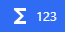Numerics can be used in event parameters. They receive a numeric value such as object position, z-order, a variable number, etc.

• Strings (also called “Text”)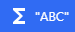Strings can be used in parameters that receive a text value. You can use this expression to read the content of a text object string, the text of another variable, etc.

## How to write an expression?

### Simple expressions: just enter a number or a text

If you don't need to do a complex calculation, you can just enter a number (for example: 123). If the expression is a string, you can enter a text surrounded by quotes (this is important).

In these examples, we're setting a bullet speed to 310 pixels per second, and setting the animation of an object to SpaceshipIdle (this could be anything else, it depends on what animations are stored in the object).

If you enter an invalid formula, for example if you forget to put double quotes around your text, the field will be red and will display the error what was found: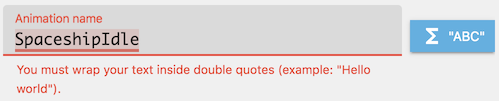### Complex expressions: use functions and operators

If you need a more complex calculation, you can use operators and functions.

• Operators are the usual mathematical operators: `+` (add), `-` (subtract), `*` (multiply) and `/` (divide). For example, you can enter “10+10”. For strings, you can only use `+` to concatenate two texts.
• Functions are values that will be computed during the game.

When you start to write a function name, GDevelop will show you a list of functions (or objects) corresponding to what your typing (this is called “smart” autocompletion). You need to enter the function name, followed by parenthesis

• Functions without objects are called with just their names: `FunctionName()`.
• Functions of objects are started with the object name and a dot: `Object.FunctionName()`.
• Finally, for objects having behavior, using a double colon (`::`) to separate the behavior name from the function: `Object.BehaviorName::PropertyName()`

For example, we can create an object at a position that is slightly to the right of another (called “EnemyBullet”) by entering these expressions: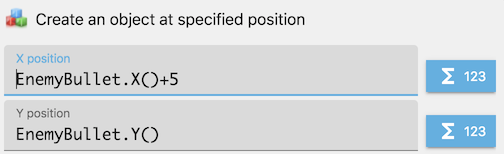## Finding functions that are useful for your game

There are a lot of functions in GDevelop. You can discover them by clicking on the blue button on the right of field where you're entering an expression. This will open a menu organised by categories, containing a list of all functions: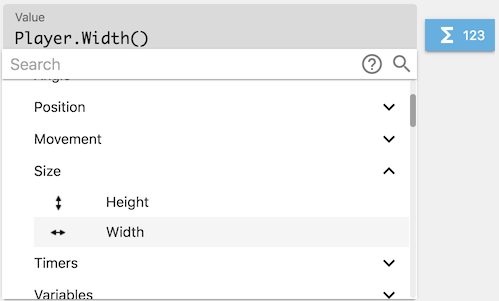When you start to write a function or object name, GDevelop will show you a list of available functions. This is called a smart auto-completion: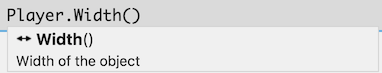Finally, you can find a list of all expressions and their descriptions here.